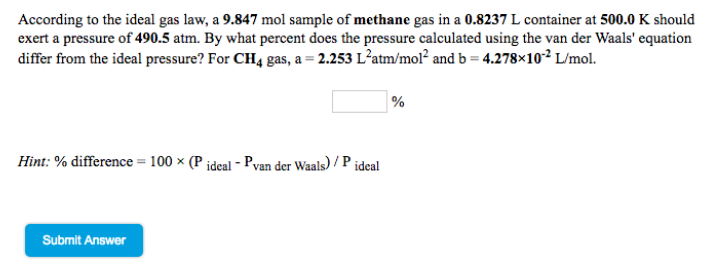# Problem: According to the ideal gas law, a 9.847 mol sample of methane gas in a 0.8237 L container at 500.0 K should exert a pressure of 490.5 atm. By what percent does the pressure calculated using the van der Waals' equation differ from the ideal pressure? For CH4 gas, a = 2.253 L2 atm/mol2 and b = 4.278 x 10-2 L/mol.

###### FREE Expert Solution
79% (275 ratings)###### Problem Details

According to the ideal gas law, a 9.847 mol sample of methane gas in a 0.8237 L container at 500.0 K should exert a pressure of 490.5 atm. By what percent does the pressure calculated using the van der Waals' equation differ from the ideal pressure? For CH4 gas, a = 2.253 L2 atm/mol2 and b = 4.278 x 10-2 L/mol.